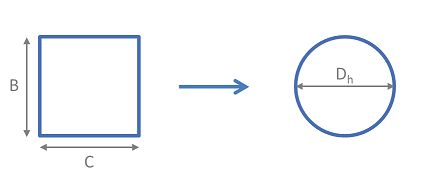# Summary

Hydraulic mean diameter provides a method by which non-circular pipe work and ducting may be treated as circular for the purpose of pressure drop and fluid flow rate calculations. This article provides the equations required to determine the hydraulic diameter for a range of non-circular geometries.

# Definitions

 : Area : Characteristic Length : Characteristic Length : Characteristic Diameter : Hydraulic Diameter : Wetted Perimeter : Hydraulic Radius : Characteristic Angle (Radians)

# Introduction

The hydraulic diameter (aka hydraulic mean diameter) is used for a fluid flowing in a pipe, duct or other conduit of any shape. This uses the perimeter and the area of the conduit to provide the diameter of a pipe which has proportions such that conservation of momentum is maintained.The concept of the hydraulic diameter allows the use of relationships developed for circular pipes with non-circular conduits. It works well for turbulent flow where geometry is less important, but should not be used for the laminar flow regime, which is influenced to a much higher degree by conduit geometry.

# Calculation of Hydraulic Diameter

The hydraulic diameter is calculated as 4 times the flow area divided by the wetted perimeter of the conduit.

# Hydraulic Diameter for Simple Geometries

This list contains the hydraulic diameter for some simple shapes.

# Usage of Hydraulic Diameter and Equivalent Diameter

## Using the Hydraulic Diameter in Pressure Loss Calculations

The hydraulic diameter may be used to determine the flow regime of a flowing fluid by calculating Reynolds Number using the hydraulic diameter. If the fluid is in the turbulent regime it may also be used to calculate the friction factor which is subsequently used to calculate the pressure loss for the system.

For the calculation of pressure loss and Reynolds Number the velocity should be calculated by dividing the volumetric flow by the actual cross sectional area of the flow path, and not by using the hydraulic diameter.

## Equivalent Diameter

An alternative to the hydraulic diameter, called the equivalent diameter was developed in 1948. The equivalent diameter aims to use empirically determined relationships between non-circular geometries and the equivalent diameter to achieve the same outcome as the hydraulic diameter.

Current research (Koch, 2008) concludes that the equivalent diameter is a poor approximation and should not be used for pressure loss calculations.

The hydraulic radius ( ) is often used for systems with free surface flows, such as water in open channels, streams or rivers. In this case the hydraulic radius is defined as the flow area divided by the wetted perimeter.

This results in the potentially confusing situation where the hydraulic diameter is not twice the hydraulic radius, but instead is 4 times the hudraulic radius.

1. Perry's Chemical Engineers' Handbook, Eighth Edition2. Chemical Engineering Volume 1, Sixth Edition: Fluid Flow, Heat Transfer and Mass Transfer (Coulson & Richardson's Chemical Engineering)